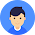# Introduction to SQL Server cube

## Introduction to SQL Server CUBE

Grouping sets specify groupings of data in a single query. For example, the following query defines a single grouping set represented as (brand):
If you have not followed the `GROUPING SETS` tutorial, you can create the `sales.sales_summary` table by using the following query:
Even though the following query does not use the `GROUP BY` clause, it generates an empty grouping set which is denoted as ().
The `CUBE` is a subclause of the `GROUP BY` clause that allows you to generate multiple grouping sets. The following illustrates the general syntax of the `CUBE`:
In this syntax, the `CUBE` generates all possible grouping sets based on the dimension columns d1, d2, and d3 that you specify in the `CUBE` clause.
The above query returns the same result set as the following query, which uses the  `GROUPING SETS`:
If you have `N` dimension columns specified in the `CUBE`, you will have 2N grouping sets.
It is possible to reduce the number of grouping sets by using the `CUBE` partially as shown in the following query:
In this case, the query generates four grouping sets because there are only two dimension columns specified in the `CUBE`.

## SQL Server `CUBE` examples

The following statement uses the `CUBE` to generate four grouping sets:
1. (brand, category)
2. (brand)
3. (category)
4. ()
Here is the output:In this example, we have two dimension columns specified in the `CUBE` clause, therefore, we have a total of four grouping sets.
The following example illustrates how to perform a partial `CUBE` to reduce the number of grouping sets generated by the query:
The following picture shows the output:In this tutorial, you have learned how to use the SQL Server `CUBE` to generate multiple grouping sets

1.2.